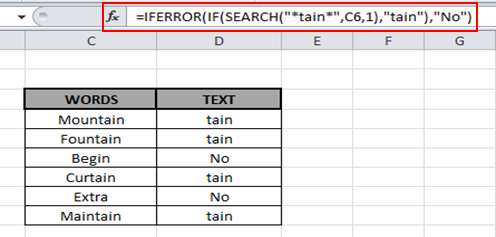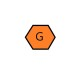# If a Cell Contains a Certain Text Then Put a Specified Text in Another Cell

In this article, we will learn how to search a certain text using Excel functions.

If you need to find a text string, Excel if cell contains text. Use a combination of IFERROR, IF and SEARCH function in Excel 2016.

IFERROR function returns a custom result if an error occurs in the formula and a standard result when no error occurs.
Syntax:

 =IFERROR (value or formula, value_if_error)

SEARCH function finds for a certain substring or value from the string or cell reference and returns the position of the first character of the certain text in the selected cell.
Syntax:

 =SEARCH (substring, string or cell reference, [start_num])

IF function is one of the logical functions, to return one value if the condition is true and another value if it's false.

Syntax:

 =IF(logical_test, [value_if_true], [value_if_false])

Let’s look at this table below, here we took some strings. We need to find a certain substring in these.Type the formula in the cell

 =IFERROR(IF(SEARCH("*tain*",C4,1),"tain"),"No")

Explanation:
Substring: tain
SEARCH function searches for the substring in the cells.
IF function checks the cell value and if it finds the substring within text it returns True else False.
IFERROR catches any error and returns No as output

Copy the formula in other cells, select the cells taking the first cell where the formula is already applied, use shortcut key Ctrl+ DAs you can see the words which contain the substring, returns the substring or else it returns No.

Hope you learned how to search with the use of SEARCH, IF and IFERROR.You can perform these tasks in Excel 2013 and 2010. Find more articles on searching text here. Please do mention your views or questions below in the comment box. We will help you.

We would love to hear from you, do let us know how we can improve, complement or innovate our work and make it better for you. Write us at info@exceltip.com

## Users are saying about us...

1.I copied the formula: =IFERROR(IF(SEARCH(“*tain*”,A2,1),”tain”),”No”)
into cell b3, and after hitting return, got an Excel error "The formula you typed contains an error."

•Hi Mike,

After copy the formula in Excel please overwrite the quotation mark (") with quotation mark (") then press enter. you will get the appropriate result.

•Julie, Mike's cousin

May I suggest that it would be helpful to explain why you advise this? The computer converted the quotation marks in your answer to "smart" quotes, so I believe the spirit of the answer was lost. In the formula on this page, the quotation marks are word processed quotations rather than the quotation mark as typed by the keyboard. Excel formulas require the regular quotation mark rather than the nice looking quotation marks. Thanks!

•Not wishing to be the only one to state the obvious, are you sure this is correct?
There is no data in cell rage A2 to A7 in the examples above.
The data is in cells A5 to A10!

2.How would I do this in a nested formula? Say if I had three categories
not 2. ie if search tain then call it 'tain', if search tra then call it tra if none found call it no

IFERROR(IF(SEARCH(“*tain*”,A2,1),”tain”),”(IF(SEARCH(“*tra*”,A2,1),”tra”),”No”)

•Hi Craig,

You can use below formula to solve your problem:-

=IF(ISNUMBER(SEARCH("tain",J7,1))=TRUE,"tain",IF(ISNUMBER(SEARCH("tra",J7,1))=TRUE,"tra","No"))

Thanks

•How would you use this formula to search a range of fields - and if any of the fields contained specified text you would return XYZ text result?

•What if you have 100's of criteria which bring up multiple regions. Would you have to do a very large formula or is there a quicker way?

•I was trying to get the formula to return three different statements but it hangs up at =IF(ISNUMBER(SEARCH(“tain”,J7,1))=TRUE,”tain”,IF(ISNUMBER(SEARCH(“tra”,J7,1))=TRUE,”tra”,”No”)) the second IF(ISNUMBER and thinks that the rest is for untrue so it just returns #NAME?. Also I would like it to return a blank cell if there is nothing in the first cell.

•Hi Eric,
You don't need True in formula. Try this formula.
=IF(ISNUMBER(SEARCH("tain",J7,1)),"tain",IF(ISNUMBER(SEARCH("tra",J7,1)),"tra",IF(ISBLANK(J7),"","No")))

•i need a formula to find out if column/row e2 contains the same text from column p2 or q2

3.Also in nested formula, I have 4 categories. If there is a prefix PSERI, it should be called Maintenance, prefix of PSI will be called Sales and SER & S-INV will be called Unposted. Is this possible and how?

4.I am trying o use the formula across sheet in a workbook but with no luck !!!
If in the sheet ISS Server Column I I have Microsoft windows server 2003 write in the sheet sheet2 the column A to I from ISS Server..

=IF(ISNUMBER(SEARCH("*Microsoft Windows Server 2003*",'ISS Servers'!H2)),'ISS Servers!H2'," ")
any idea?

Thanks,
Dom

5.What if I want to find multiple values in the original cell? Such as two years listed at different points in the text string...

6.trying to search for 3 key words within text and return the found word back to another cell...using this formula.

=IFERROR(IF(SEARCH("*FIELD*",AU11,1),"FIELD"),"(IF(SEARCH("*FCS*",AU11,1),"FCS"),"(IF(SEARCH("*CPP*",AU11,1),"CPP"),"?")

Excel won't allow it, what am I missing?

7.Stephanie Rivera

I am trying to do this in Google Sheets and it's not working. Normally they are pretty much the same with their formulas.

=IF(ISNUMBER(SEARCH(“T*”,A9,1))=TRUE,”Trial”,IF(ISNUMBER(SEARCH(“C*”,A9,1))=TRUE,”MC”))

My question is, why are you putting the 1 in there?
And also why do you think mine isn't working?
If cell A9 starts with letter T, I want the other cell to say "Trial"
If cell A9 starts with letter C, I want the other cell to say "MC"

8.Hi Guys,

In reference to the above example where the word "tain" is to be displayed, I have a list of 50-100 keywords, as in apple, orange, kiwi...etc 50-100 words.

If these keywords are found in a specific column then that keyword should be displayed in another column. Also, this list is subject to change by users as input.

I guess this can be resolved using a VBA.

9.Hello,

I have some text in one cell like (ACC_MS_21x5_INC-Rsln-P3-ERP-EBS) I put your formula =IFERROR(IF(SEARCH("P3",A1,1),”P3”),”No”) according to my need, Here I want If the cell text contains P3 then I need to put value P3 in another column. But it throwing an error. Could you please let me know issue?

•It should work. If it is returning #NAME error then Try replacing ” with " in formula. It will work.
=IFERROR(IF(SEARCH("P3",A1,1),"P3"),"No")

10.Hello.

I am trying to figure out a formula which will help me do the following: in a certain cell i would like to search a keyword only in the first 4 characters. if the keyword is found in those 4 characters, the formula will return the cell without the 4 characters.

11.Will this work in rows instead of searching in column?

12.I need to copy corresponding value of the cell

=IFERROR(IF(SEARCH(“*tain*”,A2,1),”Cell.Value(A2)”),”No”)
I need to copy the whole value of A2 in the cell.

13.i have a sentence in column DA row 1 " information is in abc.pdf" similarly in multiple rows of same column. need a macro to extract file name with .pdf of column DA

14.I have 5 sheets, 5th sheet has a list a1-a27 that have been put into a drop down list from sheets 1-4, each a1-a27 have a result listed in b1-b27. How do i create if formula for if one of the a column is listed then b column is shown in a located cell on sheets 1-4?

15.Hayley Cunningham

Can anyone help please. I am using excel to summarise contractual clauses and want to create a formula to identify if various versions of a search word are contained within a specificic cell. In the below instance I was trying to create a formula to idenfify if cell A6 contains either 'Indemnity' or indemnities:

IFERROR(IF(SEARCH("*Indemnity*",A6,1),"Yes"),"(IF(SEARCH("*Indemnities*",A6,1),"Yes"),"No")

The formula came up with an error though, is anyone able to help guide me as to where i'm going wrong please? The above formula came up with an error.

16.i need a formula to find out if column/row e2 contains the same text from column/row p2 or q2

17.Hi. I want to search a cell for multiple possible similar words, and return a value "1" if any of those words are in that text, and a value "0" if none of those words are there. EG "IF cell A2 contains Happy or Smiling or Joyful or Cheerful, then value = 1, otherwise value = 0". Is that possible? Thanks.

18.Hello, I want a text in one cell after checking three different words.
e.g. if a row contains Javier B1, Ela A1and Sam C1, I want to segregate the A1, B1 and C1 text in one cell beside them
Javier B1 B1
Ela A1 A1
Sam C1 C1
But the formula should contain the comparison for all the three variants(A1, B1, and C1)
Is it possible?
Thanks.

19.Girish kumar Metlapalli

Suppose in excel if my answer is yes then the cells selected should be able to enter any text i want to enter and,
if the answer in the cell is No, then the selected cells answer should be No.

20.Hi everyone, I have a small problem with a vba formula to create with excel.
I state that I am not able to create vba, but I was looking for information with which to be able to create it. I hope you can help me I would be really grateful.

I expose the problem:

I would like to enter a formula that:

The moment I enter a particular name in one cell, other names I decide appear in other cells. It's possible to do it?

21.I use an excel sheet for vehicle servicing. Column C shows service due date, column D shows next service date =C3+42 , How would I get c3 to show date in D3 if the letter D was inputted into cell G3

•Hi there,

You can use this formula in D3
=If(G3="D",C3+42,"")

This will only enter the formula C3+42 in D3 if the column G3 has letter D in it. Else nothing ("") will be shown in D3.

22.how so i extract data from a roster and covert it to a summarized table. For example 10 person 2 group in a duty roster. i need to convert the two roster to a summarize table on who and who on AM shift or night shift on specific date...thank you very much if you can help

23.If I wanted to find the word CRIT within column D, and if found, check to see if column J is empty, and if so, enter 99999 there, how might I accomplish that? Thanks! Your site is very helpful!

24.Hi,
How can i achieve this assuming i have a table in excel containing

Material
Transport
LAbour

Then if i have multiple figures that i consider as materials they all add up to cell of material, same case to labour and transport?

Terms and Conditions of use

The applications/code on this site are distributed as is and without warranties or liability. In no event shall the owner of the copyrights, or the authors of the applications/code be liable for any loss of profit, any problems or any damage resulting from the use or evaluation of the applications/code.